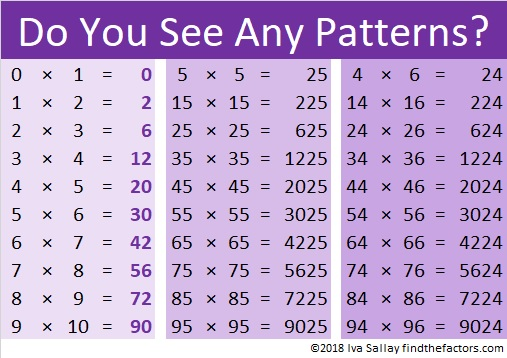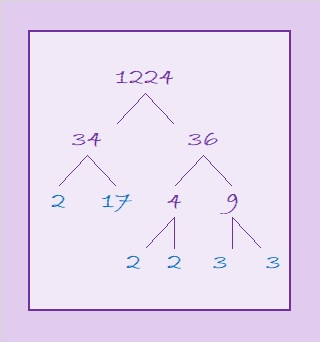# How I Knew Immediately that a Factor Pair of 1224 is . . .

Contents

### Check Out This Pattern!

12 = 3 × 4 and 24 is one less than 25. Those two facts helped me to know right away that 35² = 1225 and 34 × 36 = 1224. Study the patterns in the chart below and you will likely be able to remember all of the multiplication facts listed in it!a² – b² = (a – b)(a + b)
You may remember how to factor that from algebra class. Here when b = 1, it has a practical application that can allow you to amaze your friends and family with your mental calculating abilities!

I’ve only typed a small part of that infinite pattern chart. For example, if you know that 19 × 20 = 380, then you can also know that 195² = 38025 and 194 × 196 = 38024.

Also because of that chart, I know that 3.5² = 12.25 and 3.4 × 3.6 = 12.24
(Also (3½)² = 12¼, but 2½  × 4½ = 11¼ because 3-1 = 2, 3+1 = 4, 12-1 = 11
thus 2.5 × 4.5 = 11.25 and 2½  × 4½ = 11¼)

You could also let b = 2 so b² = 4. Then 25 – 4 = 21, and you could know facts like
33 × 37 = 1221 or 193 ×  197 = 38021.

I hope you have a wonderful time being a calculating genius!

### A Factor Tree for 1224:

When a number has many factors, I often will make a forest of factor trees for that number, but today I just want us to enjoy this one tree for 34 × 36 = 1224.### Factors of 1224:

Now I’ll share some other facts about the number 1224:

• 1224 is a composite number.
• Prime factorization: 1224 = 2 × 2 × 2 × 3 × 3 × 17, which can be written 1224 = 2³ × 3² × 17
• The exponents in the prime factorization are 2, 3 and 1. Adding one to each and multiplying we get (3 + 1)(2 + 1)(1 + 1) = 4 × 3 × 2 = 24. Therefore 1224 has exactly 24 factors.
• Factors of 1224: 1, 2, 3, 4, 6, 8, 9, 12, 17, 18, 24, 34, 36, 51, 68, 72, 102, 136, 153, 204, 306, 408, 612, 1224
• Factor pairs: 1224 = 1 × 1224, 2 × 612, 3 × 408, 4 × 306, 6 × 204, 8 × 153, 9 × 136, 12 × 102, 17 × 72, 18 × 68, 24 × 51 or 34 × 36
• Taking the factor pair with the largest square number factor, we get √1224 = (√36)(√34) = 6√34 ≈ 34.98571### Sum-Difference Puzzle:

1224 has twelve factor pairs. One of the factor pairs adds up to 145, and a different one subtracts to 145. If you can identify those factor pairs, then you can solve this puzzle!If finding a sum and a difference equalling 3-digit 145 is too challenging, the chart below will be helpful.### More about the Number 1224:

1224 is also the sum of two squares:
30² + 18² = 1224

1224 is the hypotenuse of a Pythagorean triple:
576-1080-1224 which is (8-15-17) times 72
That triple can also be calculated from 30² – 18², 2(30)(18), 30² + 18²

293 + 307 + 311 + 313 = 1224 making 1224 the sum of four consecutive prime numbers.

This site uses Akismet to reduce spam. Learn how your comment data is processed.